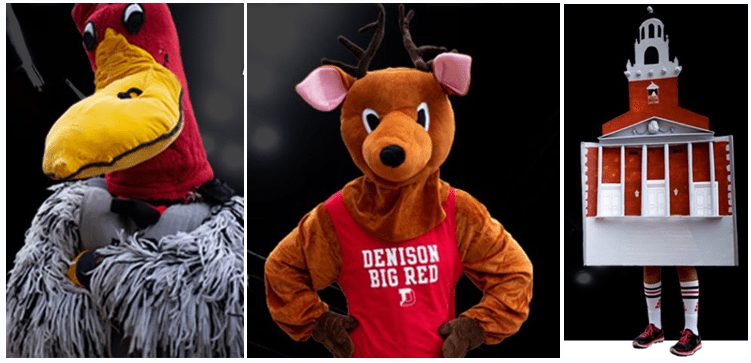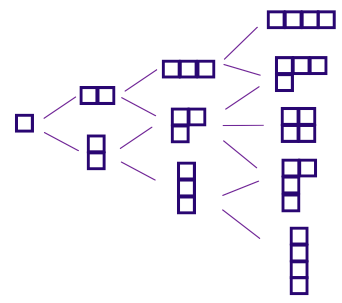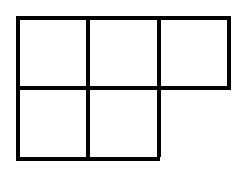Inevitably, I think back to my favorite result in mathematics: when Diaconis used the representation theory of the symmetric group to show us that psychologists just don’t get along…

Sarah Wolff
Denison University

It’s November. Here in Ohio, that means cozy sweaters, crisp mornings, pumpkin everything, Thanksgiving, and sometimes a first snow. November also means election season, and as someone who likes to think about voting I find myself lost in thought more than usual around this time.

Inevitably, election coverage in the news will start me down a rabbit hole that quickly expands to much broader scenarios than choosing a presidential candidate. You see, the type of voting that I like to think about is ranked-choice voting, aka any scenario where a group of people is asked to choose and rank from a list of items. This could be used for anything from electing a mayor to filling out a survey of preferences. So yes, I’m quickly thinking about a group of diners ranking their favorite food items. Or a group of students choosing a new Denison mascot—Buzzy the turkey vulture? Denideer the deer? Swasey the walking chapel?Mascot images from hypothetical election used courtesy of Denison University Communications.

Or if I’m in a nostalgic mood I might think about how my college soccer coach made all 20 of his players rank each other from 1 to 20. And, yes, he then shared that data with us. At the time it felt… cruel. But now that I know just how hard it really is to understand this type of data and also how hard it is for humans to handle ranking more than five choices, it feels, well, misguided.

When I think about voting, sometimes I wonder if I could get my hands on that 15+ year old data set. Sometimes I think about the incredible amount of data in this world that comes from asking people to rank their choices, and the incredible amount of variation in analyzing that data (see e.g. Bargagliotti et al.). But inevitably, I think back to my favorite result in mathematics: when Diaconis used the representation theory of the symmetric group to show us that psychologists just don’t get along. That is the result that I’d like to build to here.

## Proceeding to Choose a Procedure

Let’s back up a bit and talk about the different considerations that go into setting up an election. First and foremost: which voting method will we use? In other words: how will the voters vote? Next, which voting procedure will we use, i.e., how will a winner be determined from the votes? Once the votes are in, will we analyze that data? If so, how? Will the analysis support the outcome or point to other considerations?

Of course there is also the question of whether the voters were grouped into districts, which opens up an entire world of mathematical and moral questions (see for example the work of the MGGG Redistricting Lab).

So how will the voters vote? Well, there are many different voting methods. One is plurality voting where voters select their favorite candidate from a list (see for example the 2023 AMS Presidential election). Another, often used for electing committee members, is approval voting where voters select any candidate they approve of from a list, sometimes with a set maximum number (see for example the 2023 AMS Editorial Boards Committee election). As with all voting methods, plurality and approval voting have pros and cons. For example, approval voting could potentially create a committee entirely comprised of members reflecting the values of 51% of the electorate without a single member reflecting the values of the remaining 49%.

One voting method rising in popularity is ranked-choice voting. While its implementation in the 2021 New York City mayoral elections has made ‘ranked choice voting’ seem synonymous with ‘instant runoff voting’ (IRV), ranked choice voting is a voting method while IRV is a voting procedure. Ranked-choice voting just means the voters ranked some or all of the candidates in order of preference.

Given an election between $n$ candidates consider $S_n$, the symmetric group on $n$ letters. Each element of $S_n$ is a permutation—ranking—of the $n$ letters and corresponds to one of the choices a voter can make. We represent a ranked-choice election by a function $p:S_n\rightarrow \mathbb{C}$ where $p(\sigma)$ gives the number of voters who voted for ranking $\sigma$. In the voting theory literature $p$ is often called a profile. Note that in a typical election $p(\sigma)$ is an integer; however, working with normalized data quickly puts us outside the realm of the integers and viewing our functions with range $\mathbb{C}$ allows us to do interesting representation theory.

Here is a possible profile. (University Communications would like me to note that this is a hypothetical scenario: no such vote for a Denison mascot has happened.)

$\begin{array}{lccccr} p\large(\text{Buzzy, Denideer, Swasey})=12 &&&& p\large(\text{Buzzy, Swasey, Denideer})=7\\ p\large(\text{Denideer, Buzzy, Swasey})=22 &&&& p\large(\text{Denideer, Swasey, Buzzy})=5\\ p\large(\text{Swasey, Buzzy, Denideer})=25&&&& p\large(\text{Swasey, Denideer, Buzzy})=3 \end{array}$

Given a profile there are many different ways to select a winner. For example, we could apply a weighting vector $\mathbf{w}$ to each ranking that gives weight $w_j$ to the candidate ranked in the $j$th position. A weighting vector of $\mathbf{w}=[1,0,\dots,0]$ recovers plurality voting while $\mathbf{w}=[1,1,\dots,1,0]$ would be anti-plurality—voters indicating their least-desired candidate—and $\mathbf{w}=[n-1, n-2,\dots,1,0]$ is the well-known Borda count.

Outside of assigning weighting vectors there are plenty of other options. In 1785 the Marquis de Condorcet proposed Condorcet’s criterion: if there is a candidate that wins every head-to-head contest then that candidate should win. The 2021 New York mayoral election used instant runoff voting, which first checks if there is a candidate who is chosen in first position by more than 50% of the voters. If not, the candidate with the fewest number of votes is eliminated, the rankings are updated for the remaining candidates, and the process continues.

## Arrow Takes Aim

Different voting procedures have the potential to lead to different election outcomes. Indeed, for the profile $p$ above representing a contest between Denison mascots, plurality, Borda, and IRV each produce a different winner. So which procedure should we use?

Interestingly, that question is much harder to answer than it would seem. In 1951, economist Kenneth Arrow investigated reasonable conditions that a voting procedure should satisfy. Applied to a ranked-choice election these are:

• Unrestricted Domain: voters should be allowed to choose any ranking of the $n$ candidates.
• Pareto Principle: if all voters prefer candidate $A$ to candidate $B$, then candidate $A$ should place above candidate $B$.
• Independence of Irrelevant Alternatives (IIA): the presence of an irrelevant candidate, eg $C$, should not affect the head-to-head ranking of candidates $A$ and $B$.

Arrow then proved his impossibility theorem, often summarized as: “the only voting procedure with three or more candidates that satisfies the above conditions is a dictatorship.”

While it may seem like Arrow’s theorem declares that voting is broken, we can see that there is more to the story. The first two conditions are exceedingly reasonable but IIA is one that quickly leads to debate. A research mentor once explained IIA this way: suppose you are at a restaurant and the server tells you that you can choose between lemon meringue pie and caramel cake. You choose the cake. But then the server comes back, having remembered that they also offer ice cream sundaes. You say: “Oh! Well in that case I’ll have the pie!”Caramel cake, lemon chiffon pie, and sundae images used under CC BY 2.0.

Even with this simple example, people often come up with reasons why the interaction could make sense. And in an election, the introduction of a new, seemingly ‘irrelevant’ candidate could absolutely change some voters’ ranking of two other candidates.

Really, Arrow is telling us to be thoughtful and purposeful about elections: when choosing the voting system we will use, we need to think through which criteria are most important to us.

## Method $\neq$ Procedure $\neq$ Analysis

Think of how much ranked-choice data is out in the world. Any time you have been asked to fill out a survey of preferences, you were adding to a particular ranked-choice data set. I don’t know about you, but I’ve contributed a lot of data in my life.

Despite this sea of data, there is no standard method of analysis. Analyses vary widely but usually fall into one of three categories: descriptive methods, regression methods and clustering methods. As a simple example of a descriptive method, we could provide the average ranking of each candidate or a table of how many times each candidate is ranked in each position. A rich discussion of these three categories can be found in Bargagliotti et al., which provides examples of ranked-choice data in the fields of education and psychology, delves into how the data is traditionally analyzed and used in decision-making in these fields, and proposes new techniques that could “more fully leverage information contained in ranked data” (page 17).

To me, however, the most fascinating analysis of ranked data falls outside of the three categories above: using a generalized Fourier transform to reveal patterns within the data. To be completely honest, it can be hard to convince a non-mathematics audience to do a Fourier transform on the symmetric group to analyze their data set. The categories above are perhaps more practical—easier to explain, easier to interpret, easier to talk about with a broad audience—but the structure that a Fourier transform can reveal is just too beautiful to leave out of the conversation. My favorite example is Diaconis’s work delving into the results of the 1980 American Psychological Association (APA) presidential election.

The classical discrete Fourier transform (DFT) of a function $f:[0,1,\dots,n]\rightarrow \mathbb{C}$ is the map $f\rightarrow\{\hat{f}(k)\mid k=0,\dots,n-1\}$ arising from expressing $f$ in form:
$f=\sum_{k=0}^{n-1} \hat{f}(k)\omega_{k}.$
where $\omega_k:[0,1,\dots,n]\rightarrow \mathbb{C}$ is defined as $\omega_{k}(j)=e^{2\pi ikj/n}$. We call $\hat{f}(k)$ a Fourier coefficient.

From an algebraic perspective we see that $f$ is a function on the cyclic group $C_n$ and therefore an element of the group algebra $\mathbb{C}C_n$ of complex-valued functions on $C_n$. Taking a representation-theoretic perspective, the set of functions $\{\omega_1,\dots,\omega_n\}$ forms a complete set of inequivalent irreducible representations of $C_n$ and thus a DFT is a change-of-basis map that uses an orthogonal basis coming from the irreducible representations of $C_n$.

Taking this viewpoint allows for immediate generalization to any finite group $G$: take a function $f\in\mathbb{C}G$ and consider the Fourier coefficients that come from rewriting $f$ using a complete set of irreducible representations of $G$—an orthogonal basis for $\mathbb{C}G$. These are broad strokes: for more details see for example Section 3 of Rockmore's "Some applications of generalized FFTs" or see Crisman and Orrison for an excellent survey of representation theory applications in voting theory.

Remember how we captured election data using a profile $p$? Realizing that $p\in\mathbb{C}S_n$, we can project it into orthogonal subspaces determined by the irreducible representations of $S_n$! One reason it might make sense to use irreducible representations is because these subspaces are invariant under the action of $S_n$. This has the nice voting-theoretic interpretation that the analysis is invariant under relabeling of candidates. We definitely wouldn’t want our analysis to change if we just swapped the labels of candidates! Also, the representation theory of the symmetric group is both intrinsically beautiful and meaningful. Each irreducible representation corresponds to a partition of $n$ which can point us to relationships amongst the candidates.Using similar ideas, Diaconis took the profile $p\in\mathbb{C}S_5$ corresponding to the APA’s 1980 presidential election among 5 candidates and projected $p$ into isotypic subspaces corresponding to each irreducible representation of $S_5$. The data seemed to be concentrated in the subspace $V_3$ corresponding to the irreducible representation labeled by:This could indicate that there was a strong ‘pairs’ effect—that voters placed a pair of candidates together, either above or below the remaining three.

This observation led Diaconis to consider second-order information—positions of pairs of candidates (See Table 5 in his article “A generalization of spectral analysis with application to ranked data”). He found a large effect for ranking candidates 1 and 3 together—either both at the top or both at the bottom. It seemed that the voters either liked both or hated both. A similar effect was found for candidates 4 and 5.

Back to the APA election: at least back in 1980, the academicians and clinicians in the APA were on uneasy terms. Diaconis’s analysis captured that dynamic! That particular year, two of the candidates were academicians, two were clinicians, and one fell a bit more in the middle. "Voters seem to choose one type or the other, and then choose within, but the group effect predominates” (page 956).

This is one small example of how a seemingly obscure generalization of a Fourier transform can extract meaningful information in elections and surveys. But remember: extracting meaningful information is different from choosing a winner. While analysis may lead to considerations in choosing a voting procedure, analyzing voting data is not the same as choosing a voting procedure which is not the same as choosing a voting method.

So how should we vote? How should we analyze the votes? And how should we use this analysis? Maybe we should vote on it.

• K. J. Arrow. Social Choice and Individual Values. Yale University Press, 2012.
• A. E. Bargagliotti et al. “Using ranked survey data in education research:
Methods and applications”. In: Journal of School Psychology 85 (2021),
pp. 17–36.
• N. M. Bradburn, S. Sudman, and B. Wansick. Asking questions: The definitive
guide to questionnaire design–For market research, political polls, and social
and health questionnaires. rev. ed. San Francisco, CA: Jossey-Bass, 2004.

How hard is it to rank more than five choices?

• N. Condorcet. Essai sur l’application de l’analyse à la probabilité des décisions
rendues à la pluralité des voix. Cambridge Library Collection - Mathematics.
Cambridge University Press.
• K.-D. Crisman and M. E. Orrison. “Representation theory of the symmetric
group in voting theory and game theory”. In: Algebraic and geometric methods
in discrete mathematics. Vol. 685. Contemp. Math. Amer. Math. Soc., Providence,
RI, 2017, pp. 97–115.
• P. Diaconis. “A generalization of spectral analysis with application to ranked
data”. In: Ann. Statist. 17.3 (1989), pp. 949–979.
• J. Malkevitch, Feature Column Archive.

Includes several previous Feature Columns on the mathematics of voting and apportionment.

• D. Rockmore. “Some applications of generalized FFTs”. In: Groups and computation,
II (New Brunswick, NJ, 1995). Vol. 28. DIMACS Ser. DiscreteMath.
Theoret. Comput. Sci. Amer. Math. Soc., Providence, RI, 1997, pp. 329–369.